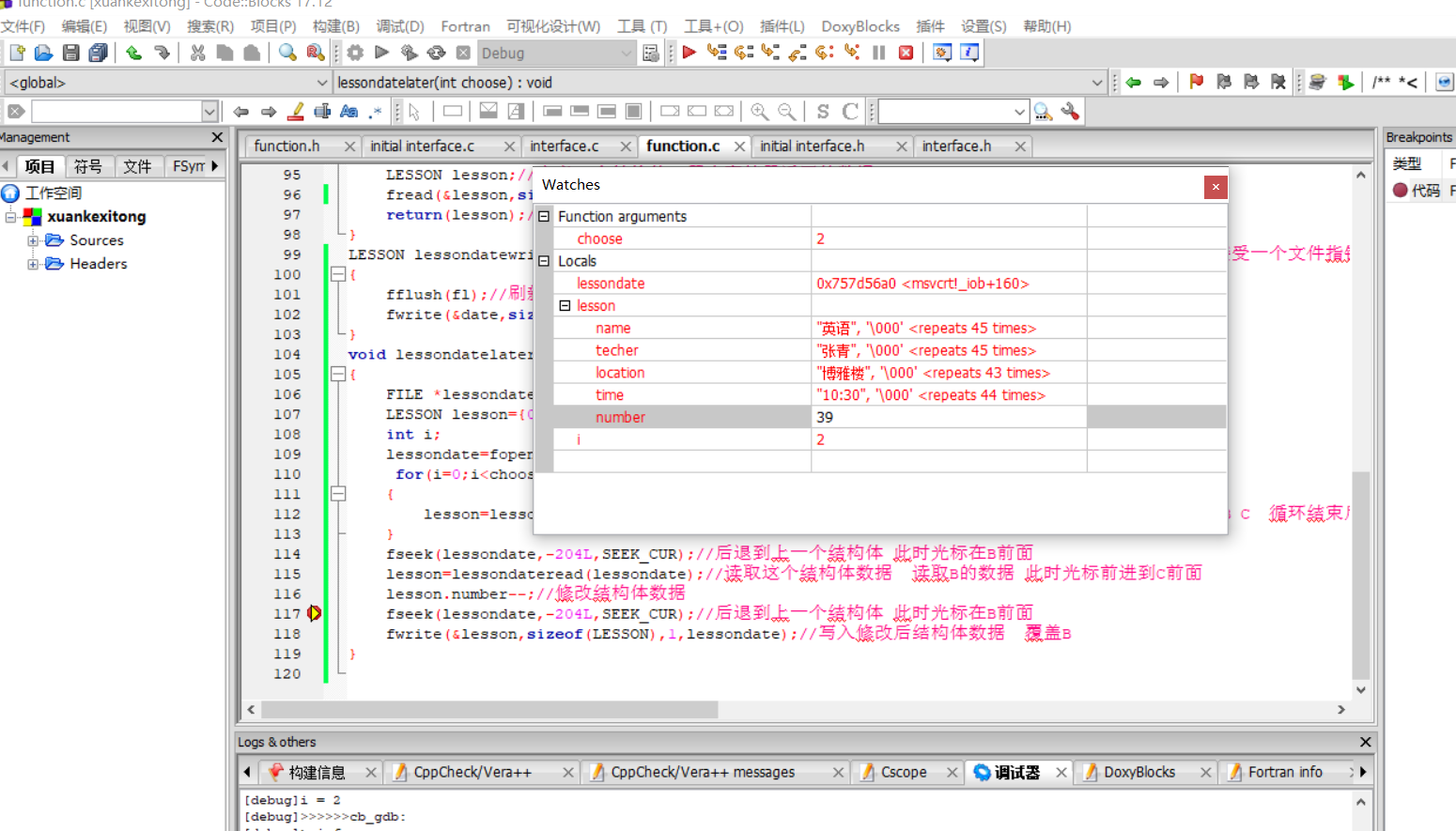C语言写入数据到二进制文件失败

# 相关代码如下

#但是lessondateltater（）调用lessondatewrite（）没写进去

# 调试结果`````` typedef struct  //定义一个Lesson类型的结构体
{
char name;
char techer;
char location;
char time;
int number;
}LESSON;
/*课程结构体参数
课程名   char    50字节
授课老师 char    50字节
位置     char    50字节
开课时间 char    50字节
座位数 int       4字节
结构体大小总计   204字节*/
LESSON lessondatewrite(FILE *fl,LESSON date)//这是一个结构体类型的函数，它可以写入课程数据，接受一个文件指针
{
fflush(fl);//刷新缓存区  使将要输入的数据写入硬盘  一次性输入大量数据时使用  避免输入失败
fwrite(&date,sizeof(LESSON),1,fl);
}
{
FILE *lessondate;
LESSON lesson={0};// 声明一个LESSON类型的变量为lesson
if((lessondate=fopen("lessondate","rb+"))==NULL)//若文件不存在，则建立文件
{
lessondate=fopen("lessondate","wb+");
//rb+打开二进制文本文件，允许读写，若不存在返回空
//wb+新建二进制文本文件，允许读写。如果文件存在则删除文件重建
}
printf("请依次输入如下数据，课程名，授课老师，教室位置，开课时间（xx月xx日xx:xx），座位数（各数据以空格隔开，回车结束）\n");
scanf("%s %s %s %s %d",&lesson.name,&lesson.techer,&lesson.location,&lesson.time,&lesson.number);
//scanf在接收字符串时  不会接收空白符（空格与回车）  逗号或其他都能接收
//%s不能使用char  而是char[]
fseek(lessondate,0L,SEEK_END);
lessondatewrite(lessondate,lesson);
fclose(lessondate);
system("cls");
}
void lessondatelater(int choose)
{
FILE *lessondate;
LESSON lesson={0};
int i;
lessondate=fopen("lessondate","rb+");
for(i=0;i<choose;i++)
{
}
fseek(lessondate,-204L,SEEK_CUR);//后退到上一个结构体 此时光标在B前面
lesson.number--;//修改结构体数据
fseek(lessondate,-204L,SEEK_CUR);//后退到上一个结构体 此时光标在B前面
lessondatewrite(lessondate,lesson);//写入修改后结构体数据  覆盖B
}

``````

1个回答